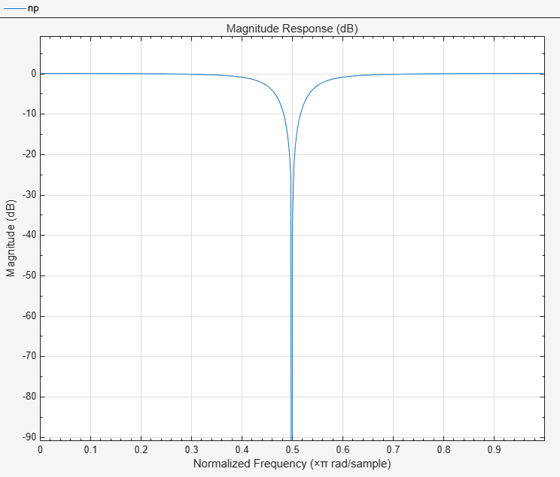# getBandwidth

Get 3 dB bandwidth

## Syntax

``BW = getBandwidth(npFilter)``

## Description

example

````BW = getBandwidth(npFilter)` returns the 3 dB bandwidth for the notch peak filter. If the `Specification` property is set to ```'Quality factor and center frequency'```, the 3 dB bandwidth is determined from the quality factor value. If the `Specification` property is set to `'Coefficients'`, the 3 dB bandwidth is determined from the `BandwidthCoefficient` value and the sample rate.```

## Examples

collapse all

Create a `dsp.NotchPeakFilter` object with the `Specification` property set to `'Quality factor and center frequency'`. The default quality factor Q is 5, and the center frequency Fc is 11,025 Hz.

`np = dsp.NotchPeakFilter('Specification','Quality factor and center frequency')`
```np = dsp.NotchPeakFilter with properties: Specification: 'Quality factor and center frequency' QualityFactor: 5 CenterFrequency: 11025 SampleRate: 44100 ```

Compute the 3 dB bandwidth of the notch peak filter using the `getBandwidth` function. The bandwidth is computed as the ratio of the center frequency and the quality factor, $\frac{\mathrm{Fc}}{\mathit{Q}}$.

`getBandwidth(np)`
```ans = 2205 ```

Visualize the filter response using `fvtool`.

`fvtool(np)`## Input Arguments

collapse all

Notch peak filter whose 3 dB bandwidth is measured, specified as a `dsp.NotchPeakFilter` object.

## Output Arguments

collapse all

3 dB bandwidth of the filter, returned as a scalar.

Data Types: `double`

### Objects

Introduced in R2014a

Watch now